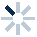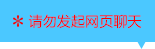| | |

||
APP端下载AndroidiPhone
|
￥158

|
3人点赞
121339人已学习
|

3天无理由退款

3天无理由退款：退款将以超级币形式退至您的超级课堂学习账户，便于您重新选购其他课程。恶意退款将被冻结账号。

• 1、介绍了$n$次方根的概念及相关概念
2、 介绍$n$次方根的两条运算性质，性质一，$(\sqrt[n]{a})^{n}=a$，当$n$为偶数时有$a\geq 0$的隐含条件。性质二，(1)当$n$为奇数时，$\sqrt[n]{a^{n}}=a$；(2)当$n$为偶数时，$\sqrt[n]{a^{n}}=\left | a \right |=\left\{\begin{matrix} a,a\geq 0\\ -a,a＜0\end{matrix}\right.$
• 1、利用根式的两条性质来进行化简时，可以由根指数$n$为偶数，被开方数$\geq 0$的隐含条件，得到$a$的范围，然后化简根式。而反过来，也可以根据化简结果来反推字母的取值范围
2、 化简根式还能帮我们解无理方程，解无理方程的基本思想就是去根号，化为有理方程
3、 化简复合二次根式$\sqrt{A\pm \sqrt{B}}$的方法：观察法、待定系数法、平方法
• 1、实数指数幂包括有理数指数幂与无理数指数幂，其中有理数指数幂包括整数指数幂与分数指数幂
2、 正数的分数指数幂是根式的另一种写法
3、 在把根式转化为分数指数幂与的过程中，如果底数对应的数或代数式是负值，则必须先利用偶次方将负数或负值代数式化为正数或正值代数式后，才能化为分数指数幂
4、 无理数指数幂是一个确定的实数，在数轴上可以找到与之对应的点
• 1、性质一，同底数幂相乘——底数不变，指数相加；性质二，幂的乘方——底数不变，指数相乘；性质三，积的乘方——等于乘方的积。其中$a> 2、 0$，$b> 3、 0$，$m$，$n\in R$
4、 性质一可以得到一个除法的推论，即$\dfrac{a^{m}}{b^{n}}=a^{m}\dfrac{1}{a^{n}}=a^{m}a^{-n}=a^{m-n}$；性质三也可以得到除法的推论，即$(\dfrac{a}{b})^{n}=\dfrac{a^{n}}{b^{n}}$
5、 一定要注意底数大于$0$的前提，如果底数是负数或负值代数式，则一定要将它转化为正数或正值代数式
• 1、学习幂运算三大性质的综合运用
2、 幂运算的三大性质在题目中的使用非常普遍，在这节课的题目中，三条性质全部都用上了，还得到一个能帮你快速解题的结论，大家要记住
• 1、对于分数指数幂$a^{\dfrac{m}{n}}$的求值，如果指数的分母$n$较大，通常我们会先将底数$a$化成某个数的$n$次方
2、 对于分数指数幂之间的乘除运算，注意两点：(1)系数与同底数幂要分开运算；(2)同底数幂相乘，指数相加；相除，指数相减
3、 运算中要注意“先化简再代入计算”的原则
• 1、学习分数指数幂运算的第三种题型，根式运算。把根式化成分数指数幂，运算起来会简单很多
2、 注意在化简多重根式时，要由内向外层层转化
• 1、学习分数指数幂运算的第四种题型：运用代数公式进行化简
2、 要熟悉三种常用的代数公式进行式子的化简
• 1、了解一个特定结构的化简结论：$(a^{n}+a^{-n})^{2}-(a^{n}-a^{-n})^{2}=4$，$a＞0$，$n\in R$
• 1、掌握指数函数的定义，注意判断指数函数的几个易错点
2、 求指数函数解析式的常用方法是待定系数法，注意不能取$x=0$，$y=1$
• 1、了解指数函数的图象与性质：底数$a$按照是否大于$1$分成两类，大于$1$时，是递增的曲线；大于$0$小于$1$则是递减的曲线
2、 它们都经过$(0,1)$这点，都一端趋近于$x$轴，一端无限上升。且$y=a^{x}$和$y=(\dfrac{1}{a})^{x}$的图象关于$y$轴对称
• 1、解决几道指数函数图象与最值函数、绝对值函数、分段函数图象结合的求值域的题目，旨在帮助大家熟悉指数函数的图象
• 1、​由单调性求字母范围时，单增推出$a$大于$1$，单减推出$0$小于$a$小于$1$
2、 注意组合函数和分段函数单调性的判断，这些内容都在之前的函数章节重点强调过的，忘记的同学记得返回观看哦
• 1、解决与值域相关的问题时，当底数固定时，根据单调性，结合图象就能求出相应区间内的值域
2、 当底数不固定，有未知字母时。有些问题不需要讨论底数，比如恒成立问题，及最值之和的问题；而有些问题需要搞清哪个是最大值，哪个是最小值，这时就要分类讨论了，比如最值之差的问题
• 1、利用指数函数的单调性解决第一类指数不等式$a^{f(x)}＞a^{g(x)}$。若$a＞1$，则指数函数$y=a^{x}$单调递增，由函数值的大小关系和指数的大小关系一致。若$0＜a＜1$，则指数函数$y=a^{x}$单调递减，由函数值的大小关系和指数的大小关系相反
2、 需要注意两点：(1)若底数不确定，需要分类讨论；(2)要化为同底数幂形式
• 1、对于第二类指数不等式$A\cdot a^{2x}+B\cdot a^{x}+C＞0$，不等式的左边可以看成是指数函数和二次函数构成的复合函数。所以可以采用换元法，将$a^{x}$整体换成$t$，化为$At^{2}+Bt+C＞0$
2、 再通过图象求$t$的范围，进而求$x$的范围。要注意一点$t＞0$
• 1、学习比较指数幂的值的大小的第一种题型——底数相同，指数不同
2、 底数相同，指数不同时，利用指数函数的单调性比较即可
• 1、学习指数幂大小比较的第二种题型——指数相同，底数不同。有两种方法：图象法或作商法
2、 图象法的基本原理是：当底数都大于$1$时，底数越大，指数函数的曲线就越陡。当底数都大于$0$小于$1$时，底数越小，指数函数的曲线就越陡。通过图象的高低就能判断出同指数时，函数值的大小了
3、 作商法，即把指数幂相除，再看指数函数的函数值是大于$1$，还是小于$1$即可
• 1、学习判断底数不同且指数不同的幂的大小的前两种常用技巧：标准值法，图象法
2、 标准值法，就是选取一个大小位于它们之间的标准值，把两个指数幂分别与这个标准值比较
3、 图象法，只要将各个图象画在同一个坐标系，然后去找相应的函数值
• 1、学习判断底数不同且指数不同的幂的大小的第三种技巧乘方化整法，即把它们同时$k$次方
2、 如果$(a^{m})^{k}＞(b^{n})^{k}$，则$a^{m}＞b^{n}$；如果$(a^{m})^{k}＜(b^{n})^{k}$，则$a^{m}＜b^{n}$。一般$k$次方后，幂会变成一个整数，所以$(a^{m})^{k}$和$(b^{n})^{k}$的大小很好比较
3、 最后一道题，滴水不漏的证明$B＞A$，难度非常大，尤其是分子分母同除以的那个式子，可谓神来之笔，大家要好好体会
• 1、指数型复合函数定义域的求法，依旧遵守由外向内的原则
2、 指数型复合函数过定点的问题有两种情况：对于外层为指数函数的复合函数，$y=a^{f(x)}$，如果$f(m)=0$，则$y=1$。即$x=m$时，$y=1$，复合函数过定点$(m,1)$。对于内层为指数函数的复合函数，即$y=f(a^{x})$，当$x=0$时，$a^{x}=1$，$y$就一定等于$f(1)$，复合函数过定点$(0,f(1))$
• 1、指数型复合函数单调性，依旧和复合函数单调性一样，通过同增异减来确定复合后整体的单调性
• 1、认识如何用定义法证明单调性。当题目要你证明单调性时，就必须用定义法来操作
• 1、介绍外层函数为指数函数的复合函数值域的求解
2、 它依旧遵循由内向外的原则。在具体求解时，可以用换元法，令内层函数为$t$。然后由$x$的范围求内层函数$t$的值域，在把$t$的值域当成外层函数定义域，求外层函数值域，即复合函数值域
3、 当指数函数的底数不确定时，要注意分类讨论
• 1、介绍内层函数为指数函数的复合函数值域的求解
2、 依旧遵循由内向外的原则。在具体求解时，可以用换元法
3、 当二次函数系数不确定时，注意分类讨论
• 1、讲解了一道指数型复合函数的综合题
2、 除了遵循了内层函数是指数函数的复合函数由内向外求值域的原则，还用到了第一类、第二类对勾函数的特性，增减性，奇偶性的知识，学员们要认真体会
• 指数及指数函数综合练习

• 1泥_巴_巴
• 2李宇霖
• 3超级学员857954
• 4超级学员
• 5超级学员1611235
• 6超级学员2065910
• 7超级学员2782293
• 8超级学员3124808
• 9超级学员3806409
• 10超级学员4105777191997人在学
￥ 78 ￥ 78122679人在学
￥ 78 ￥ 78214721人在学
￥ 0 ￥ 0121870人在学
￥ 72 ￥ 72

• 0
• 点击分享有好礼
••app端下载关注微信号